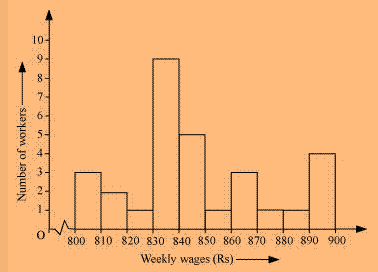# Draw a histogram for the frequency table made for the data in Question 3 and answer the following questions. (i) Which group has the maximum number of workers? (ii) How many workers earn Rs. 850 and more? (iii) How many workers earn less than Rs. 850?

Solution(i) 830-840 is the group having maximum number of workers, 9, compared to other groups.

On observing the graph we note that group having weekly wage 830-840 there are 9 workers working.

(ii) Workers earning ₹ 850 and more= 1+3+1+1+4=10

20 workers earn less than ₹ 850.

On observing the graph we note that group having weekly wage less than ₹ 850, there are 20 workers working when we add all the workers having wage less than ₹ 850.

(iii) Workers earning less than ₹ 850= 3+2+1+9+5=20

On observing the graph we note that group having weekly wage less than ₹ 850, there are 20 workers working when we add all the workers having wage less than ₹ 850.